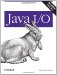# File Viewer, Part 1

I often find it useful to be able to open an arbitrary file and interpret it in an arbitrary fashion. Most commonly, I want to view a file as text, but occasionally it's useful to interpret it as hexadecimal integers, IEEE 754 floating-point data, or something else. In this book, I'm going to develop a program that lets you open any file and view its contents in a variety of different ways. In each chapter, I'll add a piece to the program until it's fully functional. Since this is only the beginning of the program, it's important to keep the code as general and adaptable as possible.

Example 4-3 reads a series of filenames from the command line in the main( ) method. Each filename is passed to a method that opens the file. The file's data is read and printed on System.out. Exactly how the data is printed on System.out is determined by a command-line switch. If the user selects text format (-a), the data will be assumed to be Latin-1 text and will be printed as chars. If the user selects decimal dump (-d), then each byte should be printed as unsigned decimal numbers between 0 and 255, 16 to a line. For example:

```000 234 127 034 234 234 000 000 000 002 004 070 000 234 127 098
```

Leading zeros maintain a constant width for the printed byte values and for each line. For hex dump format (-h), each byte should be printed as two hexadecimal digits. For example:

```CA FE BA BE 07 89 9A 65 45 65 43 6F F6 7F 8F EE E5 67 63 26 98 9E 9C
```

Hexadecimal encoding is easier, because each byte is always exactly two hex digits. The static Integer.toHexString( ) method converts each byte read into two hexadecimal digits.

Text format is the default and is the simplest to implement. This conversion can be accomplished merely by copying the input data to the console.

Example 4-3. The FileDumper program

 ```import java.io.*; import com.elharo.io.*; public class FileDumper { public static final int ASC = 0; public static final int DEC = 1; public static final int HEX = 2; public static void main(String[] args) { if (args.length < 1) { System.err.println("Usage: java FileDumper [-ahd] file1 file2..."); return; } int firstArg = 0; int mode = ASC; if (args.startsWith("-")) { firstArg = 1; if (args.equals("-h")) mode = HEX; else if (args.equals("-d")) mode = DEC; } for (int i = firstArg; i < args.length; i++) { try { if (mode == ASC) dumpAscii(args[i]); else if (mode == HEX) dumpHex(args[i]); else if (mode == DEC) dumpDecimal(args[i]); } catch (IOException ex) { System.err.println("Error reading from " + args[i] + ": " + ex.getMessage( )); } if (i < args.length-1) { // more files to dump System.out.println(" -------------------------------------- "); } } } public static void dumpAscii(String filename) throws IOException { FileInputStream fin = null; try { fin = new FileInputStream(filename); StreamCopier.copy(fin, System.out); } finally { if (fin != null) fin.close( ); } } public static void dumpDecimal(String filename) throws IOException { FileInputStream fin = null; byte[] buffer = new byte; boolean end = false; try { fin = new FileInputStream(filename); while (!end) { int bytesRead = 0; while (bytesRead < buffer.length) { int r = fin.read(buffer, bytesRead, buffer.length - bytesRead); if (r == -1) { end = true; break; } bytesRead += r; } for (int i = 0; i < bytesRead; i++) { int dec = buffer[i]; if (dec < 0) dec = 256 + dec; if (dec < 10) System.out.print("00" + dec + " "); else if (dec < 100) System.out.print("0" + dec + " "); else System.out.print(dec + " "); } System.out.println( ); } } finally { if (fin != null) fin.close( ); } } public static void dumpHex(String filename) throws IOException { FileInputStream fin = null; byte[] buffer = new byte; boolean end = false; try { fin = new FileInputStream(filename); while (!end) { int bytesRead = 0; while (bytesRead < buffer.length) { int r = fin.read(buffer, bytesRead, buffer.length - bytesRead); if (r == -1) { end = true; break; } bytesRead += r; } for (int i = 0; i < bytesRead; i++) { int hex = buffer[i]; if (hex < 0) hex = 256 + hex; if (hex >= 16) System.out.print(Integer.toHexString(hex) + " "); else System.out.print("0" + Integer.toHexString(hex) + " "); } System.out.println( ); } } finally { if (fin != null) fin.close( ); } } } ```

When FileDumper is used to dump its own .class file in hexadecimal format, it produces the following output:

```D:JAVAioexamples4> java FileDumper -h FileDumper.class
ca fe ba be 00 00 00 2e 00 78 0a 00 22 00 37 09 00 38 00 39 08 00 3a 0a
00 3b 00 3c 08 00 3d 0a 00 3e 00 3f 08 00 40 0a 00 3e 00 41 08 00 42 0a
00 21 00 43 0a 00 21 00 44 0a 00 21 00 45 09 00 38 00 46 08 00 47 07 00
48 0a 00 0f 00 49 0a 00 4a 00 4b 07 00 4c 0a 00 3b 00 4d 0a 00 0f 00 4e
...
```

In later chapters, I'll add a graphical user interface and many more possible interpretations of the data in the file, including floating-point, big- and little-endian integer, and various text encodings.Java I/O
ISBN: 0596527500
EAN: 2147483647
Year: 2004
Pages: 244